Like   Tweet   Pin   +1   inPlease keep all those impacted by 911 in prayer on this day. #neverforget

 table div table+table+table div table{width:100%;padding:0}table div table+table+table div table img{width:96.23%;padding:0;float:none}table div table+table+table div table td{width:100%;padding:0 1.88% 18px}/* styles *//* styles */ September 11th, 2020
 /* styles */ Dear Fellow Canadians, Thank you for your ongoing engagement in things that matter to the future of our nation. We are living in unprecedented and fast-paced times on the political front. The CPC leadership race ended August 24th, and the CPC leadership team and shadow cabinet was just named. In addition, Prime Minister Trudeau has prorogued Parliament and will give his next Speech from the Throne on September 23rd. If the opposition votes against it, we could be in an election this fall. We will do our best to keep you up to date on what is happening and what we can do to make a positive impact in this important season. In the meantime, we have some very interesting information to share.
 table div table+table+table+table+table+table div table{width:100%;padding:0}table div table+table+table+table+table+table div table img{width:96.23%;padding:0;float:none}table div table+table+table+table+table+table div table td{width:100%;padding:0 1.88% 18px}/* styles */table div table+table+table+table+table+table+table+table div table{width:100%;padding:0}table div table+table+table+table+table+table+table+table div table img{width:96.23%;padding:0;float:none}table div table+table+table+table+table+table+table+table div table td{width:100%;padding:0 1.88% 18px}/* styles */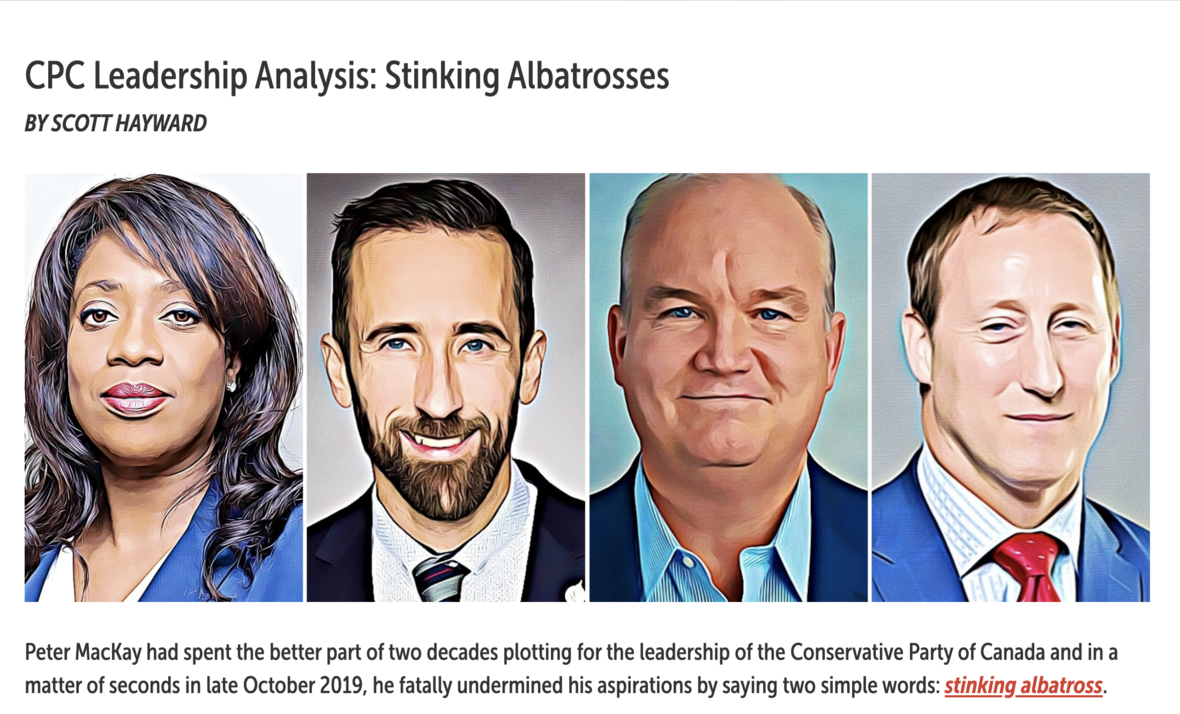## RIGHT NOW, BRILLIANT BREAKDOWN OF CPC LEADERSHIP RACE RESULTS:

Scott Hayward, from Right Now, wrote a detailed analysis of the CPC leadership race. We were so impressed that we wanted to share it with you!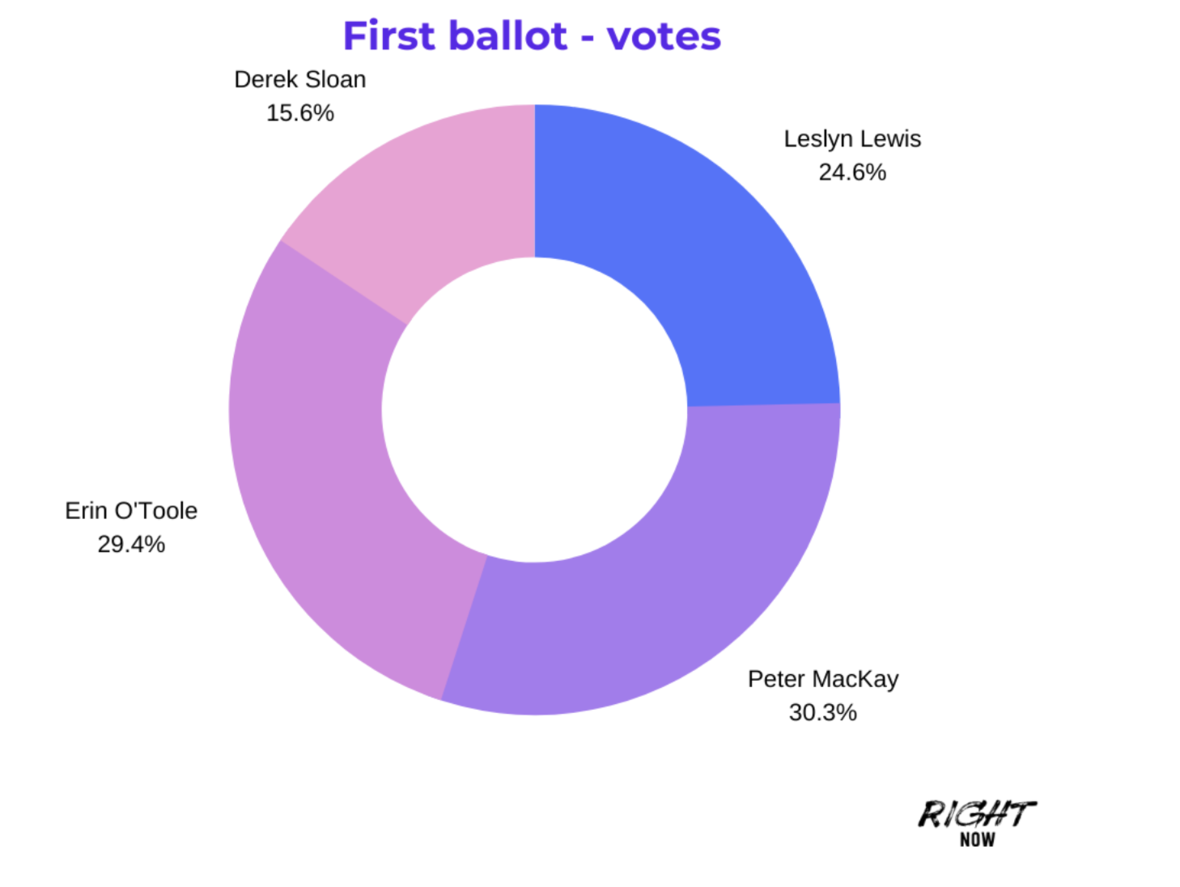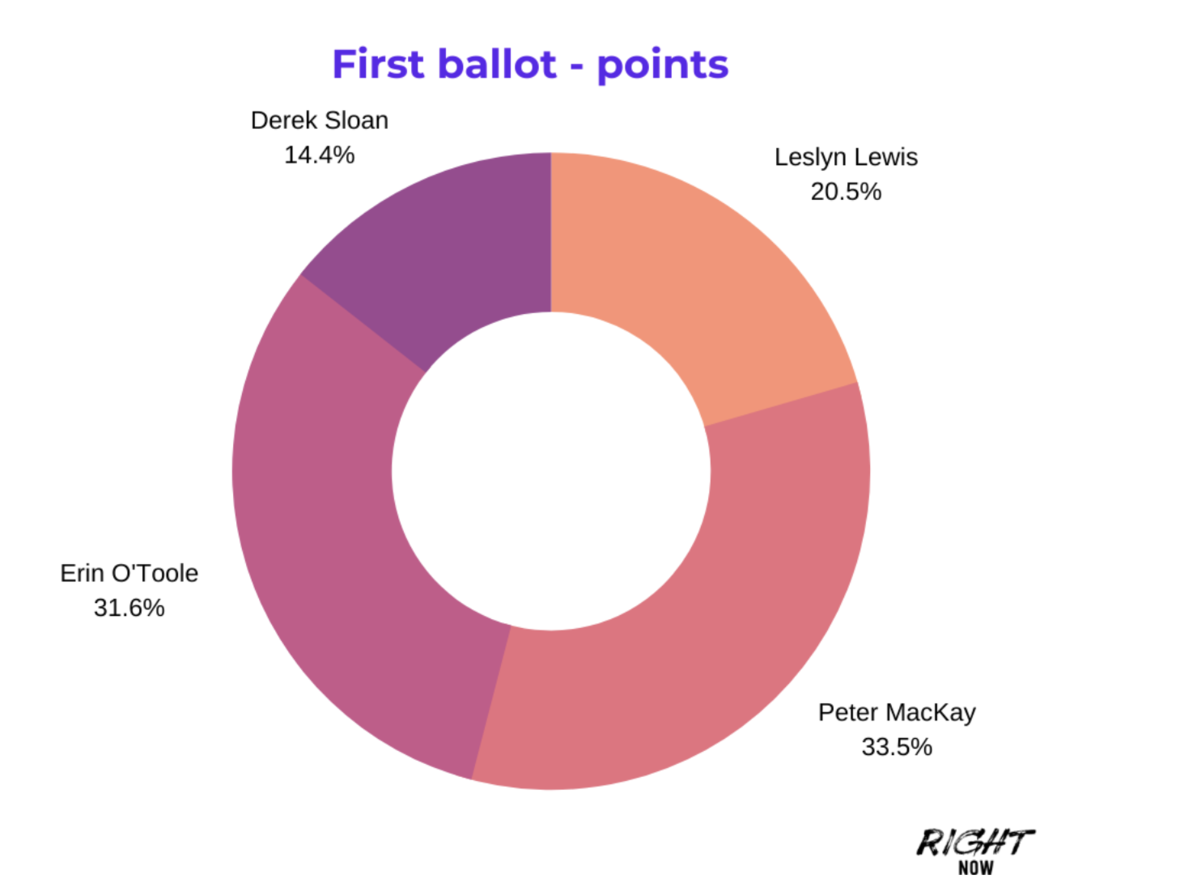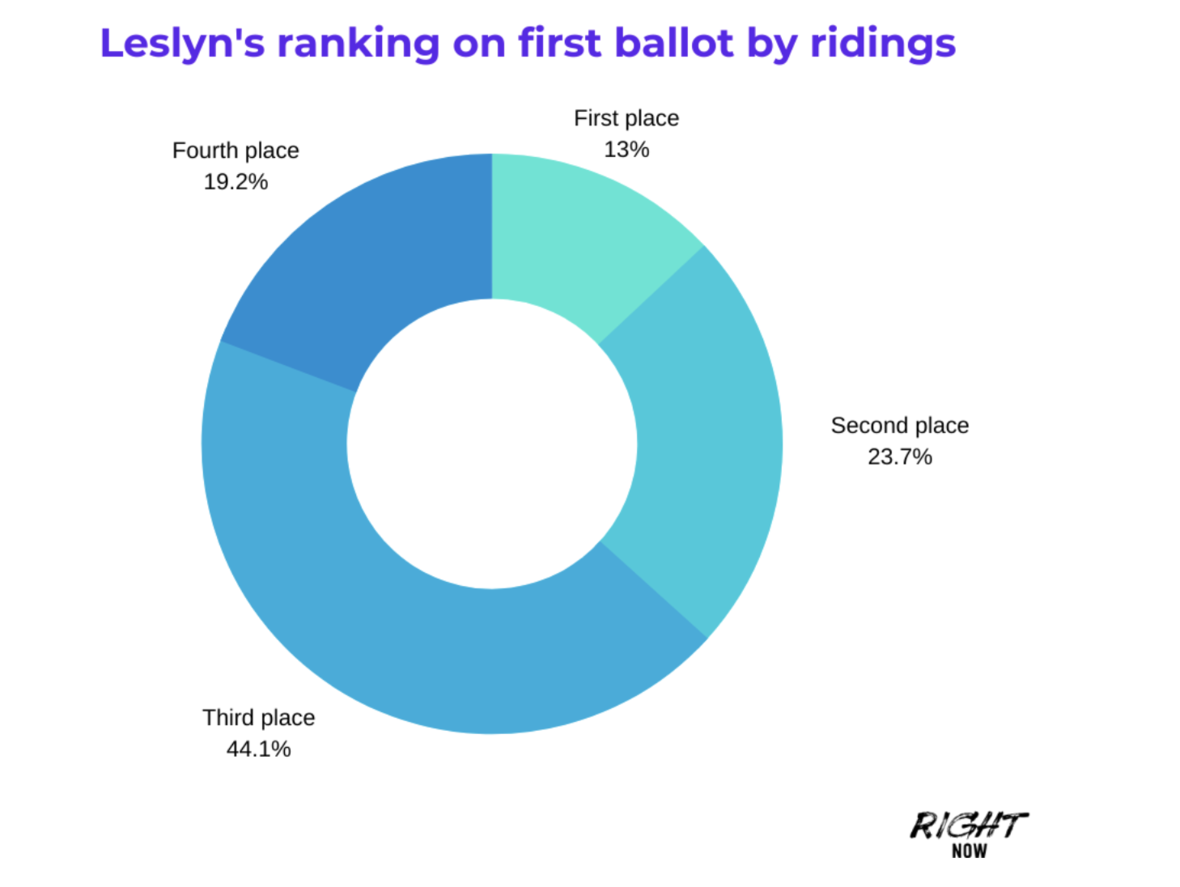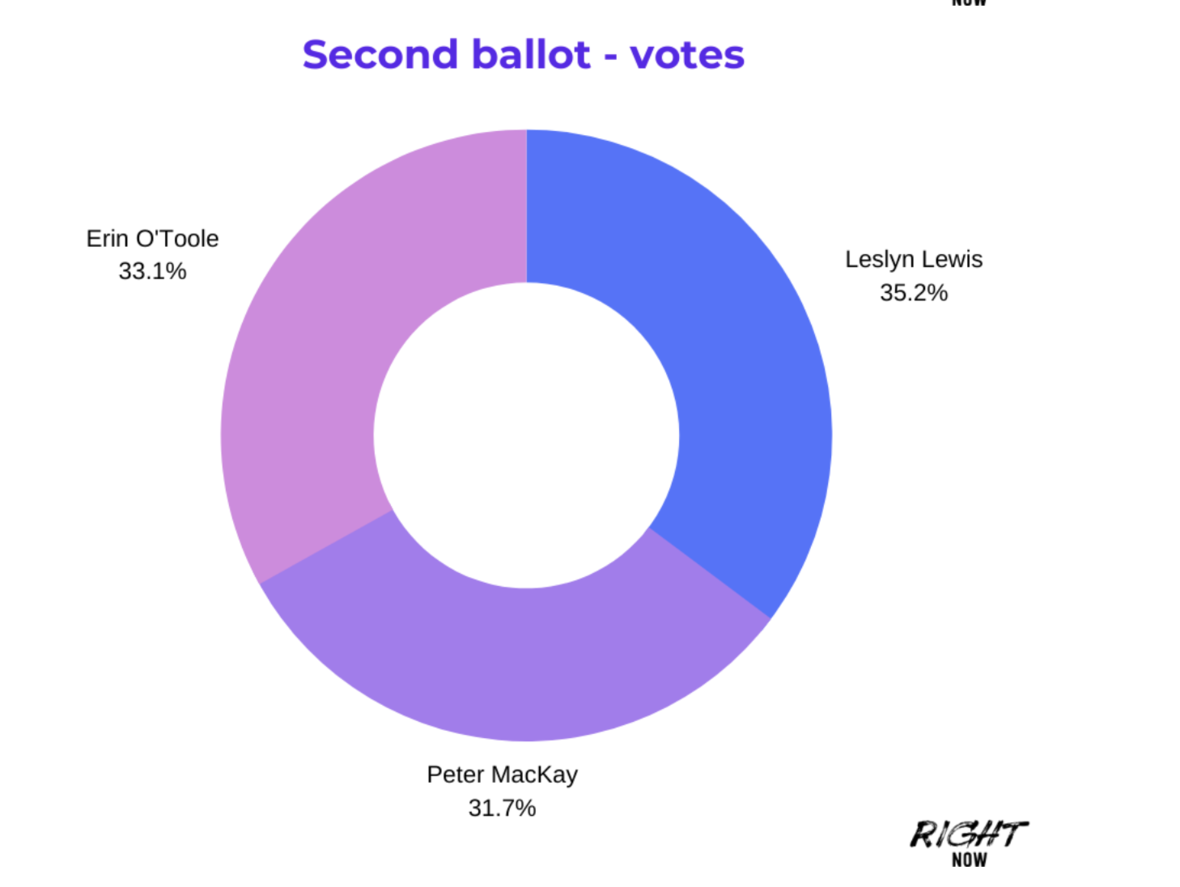Earlier this week, Conservative Leader, The Hon. Erin O’Toole, announced his new House of Commons Leadership Team and Shadow Cabinet Members with remarks that "We are here to fight for you and your family".

* The Hon. Candice Bergen, Deputy Leader

* The Hon. Pierre Poilievre, Finance
* The Hon. Michelle Rempel-Garner, Health
* Rosemarie Falk, Seniors
* The Hon. Rob Moore, Justice and Attorney General of Canada

...and others...

This is the team: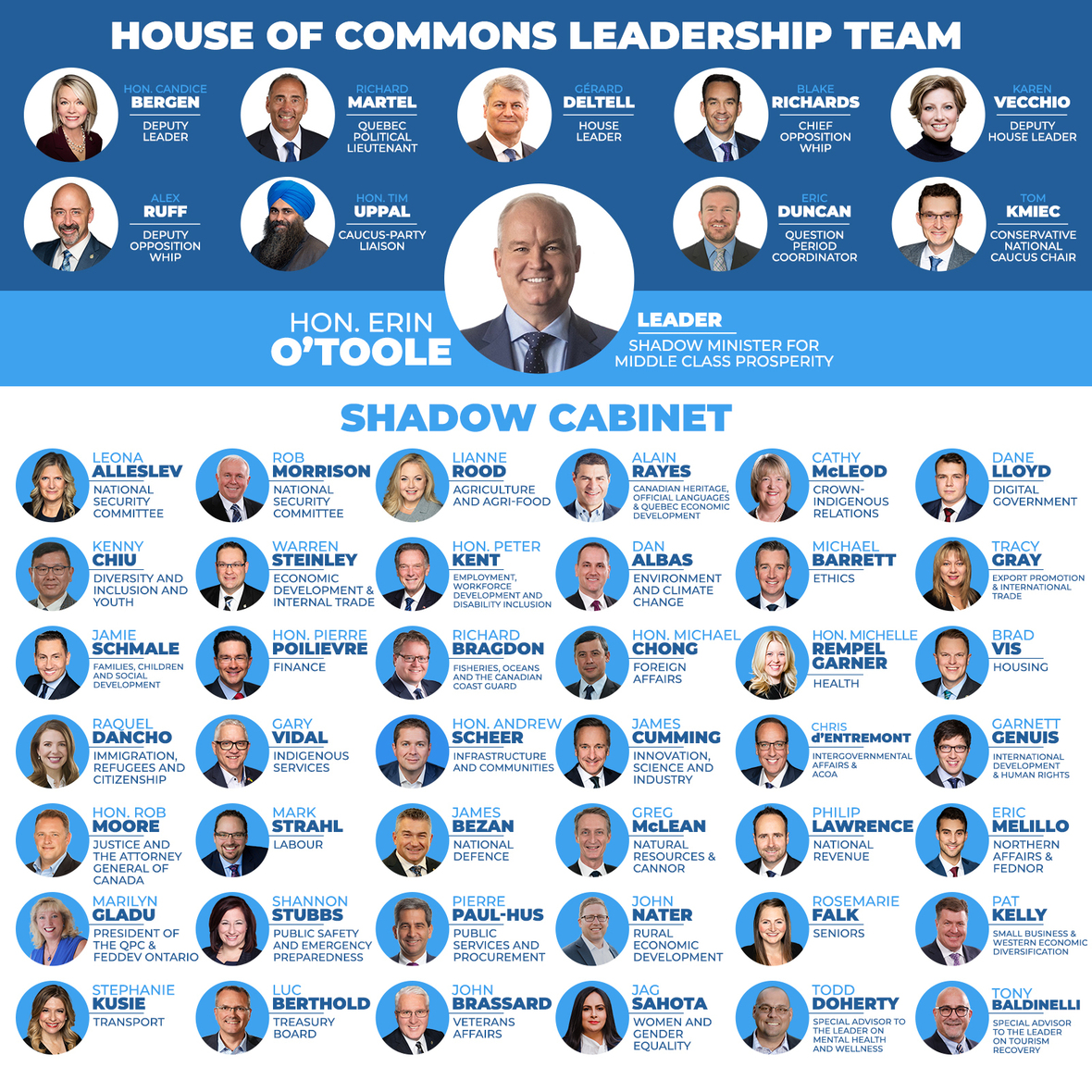▪ For the Hon. Erin O’Toole, his new House of Commons Leadership Team and the new Shadow Cabinet as Parliament returns in just a few weeks.
 ▪ For the Hon. Erin O’Toole, his new House of Commons Leadership Team and the new Shadow Cabinet as Parliament returns in just a few weeks.

## MORE ANALYTICS OF THE CPC LEADERSHIP RESULTS

In the video below, our founder, Faytene Grasseschi and Doug Sharpe from the National Leadership Briefing posted a CPC Leadership Race Reflection Video where they breakdown:

▪ The major wins for the faith and family vote
▪ The things that can be learned
▪ What we can do from this point to impact Canada
 ▪ The major wins for the faith and family vote
 ▪ The things that can be learned
 ▪ What we can do from this point to impact Canada

This is a Facebook video, if you don't have Facebook, you can watch it here on YouTube.

 table div table+table+table+table+table+table+table+table+table+table+table+table+table+table+table+table+table+table div table td,table.module-17{width:100%;padding:0}table div table+table+table+table+table+table+table+table+table+table+table+table+table+table+table+table+table+table div table{width:100%;float:none;margin-left:auto;margin-right:auto;padding:0}table div table+table+table+table+table+table+table+table+table+table+table+table+table+table+table+table+table+table div table a{border:0 none;text-decoration:none}table div table+table+table+table+table+table+table+table+table+table+table+table+table+table+table+table+table+table div table img{width:100%!important;border:0 none;text-decoration:none}/* styles */
 table div table+table+table+table+table+table+table+table+table+table+table+table+table+table+table+table+table+table+table div table{width:100%;padding:0}table div table+table+table+table+table+table+table+table+table+table+table+table+table+table+table+table+table+table+table div table img{width:96.23%;padding:0;float:none}table div table+table+table+table+table+table+table+table+table+table+table+table+table+table+table+table+table+table+table div table td{width:100%;padding:0 1.88% 18px}/* styles */table div table+table+table+table+table+table+table+table+table+table+table+table+table+table+table+table+table+table+table+table div table{width:100%;padding:0}table div table+table+table+table+table+table+table+table+table+table+table+table+table+table+table+table+table+table+table+table div table img{width:96.23%;padding:0;float:none}table div table+table+table+table+table+table+table+table+table+table+table+table+table+table+table+table+table+table+table+table div table td{width:100%;padding:0 1.88% 18px}/* styles */table div table+table+table+table+table+table+table+table+table+table+table+table+table+table+table+table+table+table+table+table+table+table div table td,table.module-21{width:100%;padding:0}table div table+table+table+table+table+table+table+table+table+table+table+table+table+table+table+table+table+table+table+table+table+table div table{width:100%;float:none;margin-left:auto;margin-right:auto;padding:0}table div table+table+table+table+table+table+table+table+table+table+table+table+table+table+table+table+table+table+table+table+table+table div table a{border:0 none;text-decoration:none}table div table+table+table+table+table+table+table+table+table+table+table+table+table+table+table+table+table+table+table+table+table+table div table img{width:100%!important;border:0 none;text-decoration:none}/* styles */
 table div table+table+table+table+table+table+table+table+table+table+table+table+table+table+table+table+table+table+table+table+table+table+table+table div table{width:100%;padding:0}table div table+table+table+table+table+table+table+table+table+table+table+table+table+table+table+table+table+table+table+table+table+table+table+table div table img{width:96.23%;padding:0;float:none}table div table+table+table+table+table+table+table+table+table+table+table+table+table+table+table+table+table+table+table+table+table+table+table+table div table td{width:100%;padding:0 1.88% 18px}/* styles */